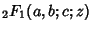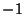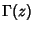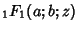## Kummer's Formulas

Kummer's first formula is(1)

whereis the Hypergeometric Function with,,, ..., andis the Gamma Function. The identity can be written in the more symmetrical form as(2)

whereandis a positive integer. Ifis a negative integer, the identity takes the form(3)

(Petkovsek et al. 1996).

Kummer's second formula is(4)

whereis the Confluent Hypergeometric Function and,,, ....

References

Petkovsek, M.; Wilf, H. S.; and Zeilberger, D. A=B. Wellesley, MA: A. K. Peters, pp. 42-43 and 126, 1996.